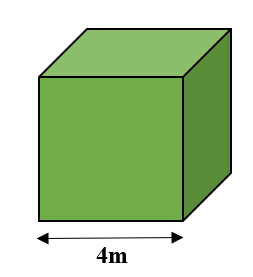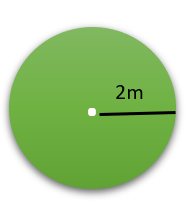GeeksforGeeks App
Open AppBrowser
Continue

# Volume Charge Density Formula

Mensuration is the branch of mathematics that deals with the measurements of geometric figures such as length, width, area, perimeter, volume etc. The geometrical figures exist in two dimensional (2D) and three dimensional (3D) forms such as Square, Cylinder, Cube, Rectangle etc.

In electromagnetism, the charge density specifies how much charge is present in given properties of the figure i.e., length, area, volume etc. Volume Charge Density specifies how much amount of charge is present in the given volume. The electric charge can be represented by the Greek symbol pho (ρ). The formula to find the volume charge density is given by-

Volume Charge density (ρ) = Charge (C)/Volume (V)

ρ = C/V

where,

ρ is charge density.

C represents charge.

V is the volume in m3.

### Sample Questions

Question 1: Find the volume charge density for the given charge and the volume are 8C and 12m3 respectively.

Solution:

Given,

Charge (C)=8

Volume (V)=12m3

Volume Charge Density (ρ)=C/V

= 8/12

= 0.66

Volume Charge Density for given data is 0.66 C/m3

Question 2: Find the volume charge density for the given charge and the volume is 18C and 9m3 respectively.

Solution:

Given,

Charge (C) = 18

Volume (V) = 9m3

Volume Charge Density (ρ) = C/V

= 18/9

= 2

Volume Charge Density for given data is 2 C/m3

Question 3: Find the charge for the given volume density charge and volume are 3 C/m3 and 6m3 respectively.

Solution:

Given,

Volume charge density (ρ)=3 C/m3

Volume (V)=6m3

Volume Charge Density (ρ)=C/V

Charge (C) = ρ×V

= 3×6

= 18

Charge for the given data is 18 C.

Question 4: Find the charge for the given volume density charge and volume are 2 C/m3 and 10m3 respectively.

Solution:

Given,

Volume charge density (ρ)=3 C/m3

Volume (V)=6m3

Volume Charge Density (ρ)=C/V

Charge (C) = ρ×V

= 2×10

= 20

Charge for the given data is 20 C.

Question 5: Find the Volume charge density for the given cube with side length 4m and the charge is 150C.Solution:

Given

Side length of cube = 4m

Volume of cube = side3

V = 43

= 64

Charge (C) = 150

Volume Charge Density (ρ) = C/V

= 150/64

= 2.34

Volume Charge Density for given data is 2.34 C/m3

Question 6: Find the Volume charge density for the given sphere with a radius of 2m and the charge is 50C.Solution:

Given

Volume of sphere = (4/3)πr3

V = (4/3)π23

= (4×22×8)/(7×3)

= 704/21

= 33.52

Charge (C) = 50

Volume Charge Density (ρ) = C/V

= 50/33.52

= 1.49

Volume Charge Density for given data is 1.49 C/m3

My Personal Notes arrow_drop_up
Related Tutorials